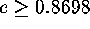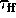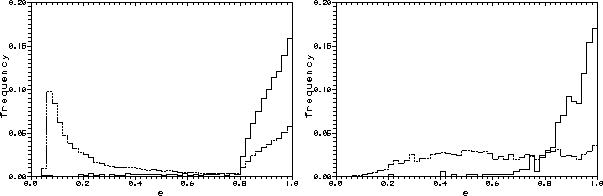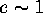Next: The ``Reflection'' Effect Up: Ejectors in Massive Binary Previous: The Radiopulsar PSRB1259-63Ā- a

## Why must a radiopulsar coupled with an optical star have high eccentricity?

The radiopulsar PSRB1259-63 in a massive binary has an extremely high eccentricity(Johnston et al., 1994). The probability of finding a pulsar in an orbit with this eccentricity is at least0.77. The natural questions ariseĀ- how such eccentricity could be explained within the framework of the theory of binary evolution and what is the probability of finding a radiopulsar in such an elongated orbit? To answer these questions we have numerically simulated the evolution of an ensemble of binaries with the aim to calculate the eccentricity distribution of PSR+I binaries. Three factors are known to affect the orbital motion of a pulsar in a binary after the supernova (SN) explosion: (a)Āthe initial eccentricity of the supernova progenitor system; (b)Āthe mass loss during SN explosion; and (c)Āthe additional ``kick'' velocityĀ of a NS caused by anisotropyĀ of collapse. All these factors were taken into account in our simulations.

The first factorĀ- the initial eccentricityĀ- is of importance only in wide systems, because mass exchange leads to orbital circularization during of the common envelopeĀ stage, so that such systems ``forget'' the initial eccentricity. Mass lossĀ associated with the SN explosion leads to a decrease of the gravitational binding energy of the binary, and the disruption of the binary occurs if the mass lost exceeds half of the total binary mass prior to the explosion. We have assumed that explosion occurs at a random moment on the orbit and all the mass thrown off by a star instantly leaves the system because the characteristic velocity of matter from the exploded star is much higher than the orbital velocity. The third factorĀ- an additional ``kick''Ā- is the most uncertain parameter, this could dramatically change destiny of the system .

We have calculated the distribution of eccentricities of binary radiopulsars with normal stars in two casesĀ- with an additional ``kick'' velocity 75 km sĀ and without it. The calculation has been done for all pulsars in pairs with normal stars and separately for visible pulsars (the optical depthfor free-free absorption in the stellar wind is less than 1). These distributions are presented in FigureĀ32.

ĀFigure 32: Distribution of binary NS with normal components over orbital eccentricities for ejectors (dashed line) and visible pulsars (solid line) without (left-hand panel) and with ``kick'' velocity 75 km s(right-hand panel) (Lipunov et al., 1994a).ĀĀ

In the case of the scenario without collapse anisotropy, the systems show a bimodal eccentricity distribution with two maximaĀ- close to 0 and at 1.

The systems with highly eccentric orbit origin from essentially wide pairs. Almost all wide systems are disrupted after the first SN explosion (formal eccentricity is more than 1) and only a few (which could have weak mass exchange) remain in binaries. On the other hand, close binaries with originally high mass ratio (q > 3) remain on almost circular orbitĀ- the primary star10-20loses a significant part of its mass during the mass exchange stage and before the SN explosion its mass is less than that of the secondary component. So the total loss due to explosion is less than a quarter of the total mass and the system remains in an almost circular orbit. If the mechanism of an additional ``kick'' is taken into account the distribution of eccentricities is almost uniform (FigureĀ32). However the distribution of systems with ``visible'' pulsars displays only one maximum atbecause the pulsars with high eccentricity are in systems with relatively large semi-major axes (so that). Moreover, these pulsars spend almost all the time at the most distant part of the orbit, which increases the probability of their detection. The probability of finding a pulsar in an orbit with the eccentricityis at least77 percent.Next: The ``Reflection'' Effect Up: Ejectors in Massive Binary Previous: The Radiopulsar PSRB1259-63Ā- a

Mike E. Prokhorov
Sat Feb 22 18:38:13 MSK 1997